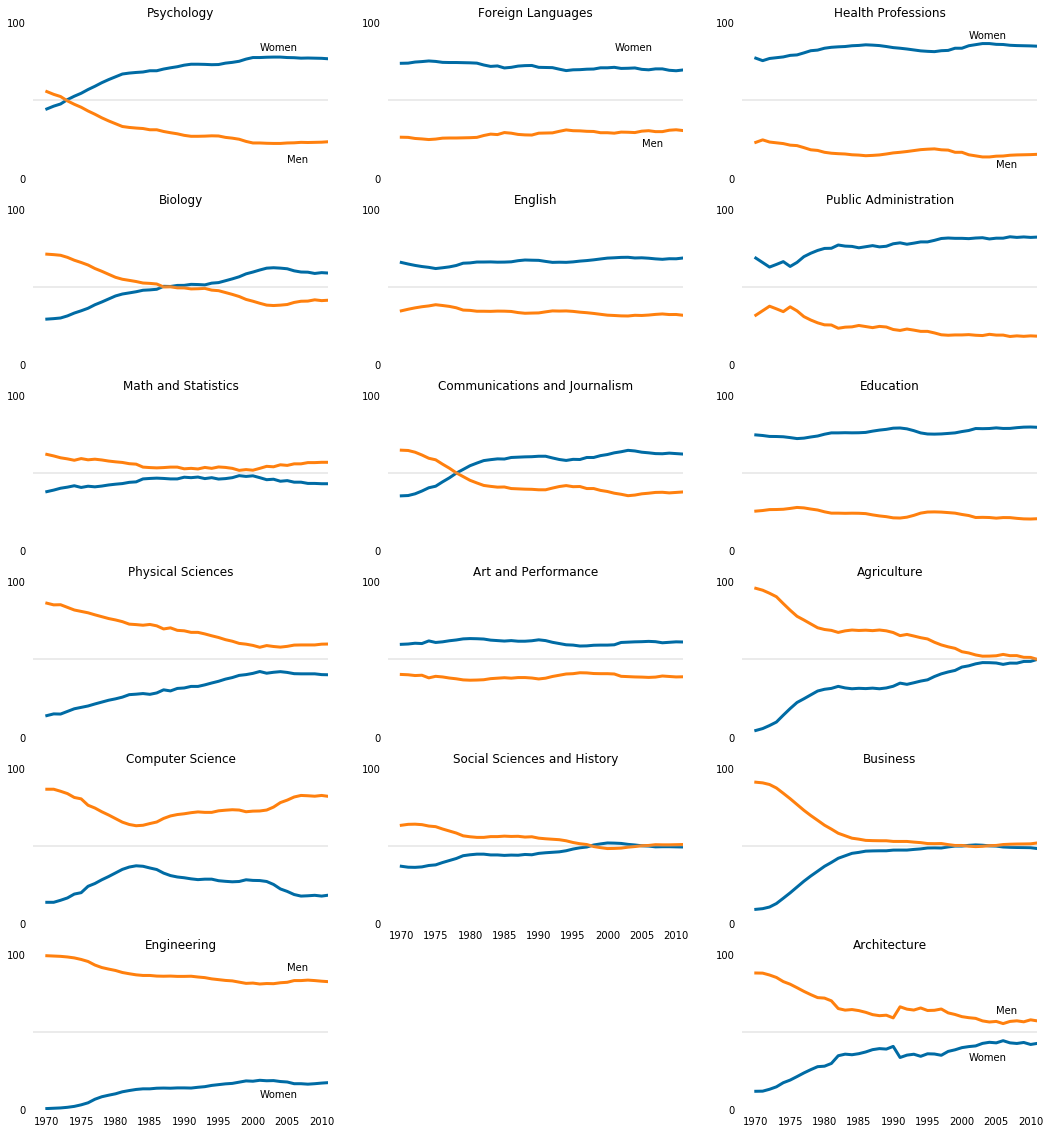First, I would like to point out that this project has nothing to do with the gender wage gap. We are simply going to look at the number of women in various majors between 1968-2011. We will then plot our findings to see if there are majors with less women than others. We'll focus on plotting asthetics in this project.

We will work with matplotlib.pyplot and we will not need the jupyter magic %matplotlib inline

In :
import pandas as pd
import matplotlib.pyplot as plt

#Set plot line colors to colorblind mode
cb_dark_blue = (0/255,107/255,164/255)
cb_orange = (255/255, 128/255, 14/255)

#Create 3 categories of majors
stem_cats = ['Psychology', 'Biology', 'Math and Statistics', 'Physical Sciences', 'Computer Science', 'Engineering', 'Computer Science']
lib_arts_cats = ['Foreign Languages', 'English', 'Communications and Journalism', 'Art and Performance', 'Social Sciences and History']


We have to think about how we are going to put our plots together. Since there are three main categories we want to look at, we will put stem_cats in one column, lib_arts_cats in another column, and finally other_cats in the last_column.

When we present our findings we want to have least amount of clutter as possible.

In :
fig = plt.figure(figsize=(18, 20))

#for stem, loop 6 times for 6 plots.
for sp in range(0,6):
#We are going to have 17 plots total, so we'll layout our plots in a 6 rows 3 columns format.
ax.plot(women_degrees['Year'], women_degrees[stem_cats[sp]], c=cb_dark_blue, label='Women', linewidth=3)
ax.plot(women_degrees['Year'], 100-women_degrees[stem_cats[sp]], c=cb_orange, label='Men', linewidth=3)

#remove the spines
ax.spines["right"].set_visible(False)
ax.spines["left"].set_visible(False)
ax.spines["top"].set_visible(False)
ax.spines["bottom"].set_visible(False)

#set x,y limits
ax.set_xlim(1968, 2011)
ax.set_ylim(0,100)

#set title
ax.set_title(stem_cats[sp])

#remove ticks marks
ax.tick_params(bottom="off", top="off", left="off", right="off")

#set tick values
ax.set_yticks([0,100])

#adds a horizontal line at y=50 position
ax.axhline(50, c=(171/255, 171/255, 171/255), alpha=0.3)

#add text to the plots at the given positions
if sp != 5:
ax.tick_params(labelbottom='off')
if sp == 0:
ax.text(2001, 82, 'Women')
ax.text(2005, 10, 'Men')
elif sp == 5:
ax.text(2005, 90, 'Men')
ax.text(2001, 8, 'Women')

#for lib arts, loop 5 times for 5 plots
for sp in range(0,5):
ax.plot(women_degrees['Year'], women_degrees[lib_arts_cats[sp]], c=cb_dark_blue, label='Women', linewidth=3)
ax.plot(women_degrees['Year'], 100-women_degrees[lib_arts_cats[sp]], c=cb_orange, label='Men', linewidth=3)
ax.spines["right"].set_visible(False)
ax.spines["left"].set_visible(False)
ax.spines["top"].set_visible(False)
ax.spines["bottom"].set_visible(False)
ax.set_xlim(1968, 2011)
ax.set_ylim(0,100)
ax.set_title(lib_arts_cats[sp])
ax.tick_params(bottom="off", top="off", left="off", right="off")
ax.set_yticks([0,100])
ax.axhline(50, c=(171/255, 171/255, 171/255), alpha=0.3)
if sp != 4:
ax.tick_params(labelbottom='off')
if sp == 0:
ax.text(2001, 82, 'Women')
ax.text(2005, 20, 'Men')

#for other categories, loop 6 times for 6 plots
for sp in range(0, 6):
ax.plot(women_degrees['Year'], women_degrees[other_cats[sp]], c=cb_dark_blue, label='Women', linewidth=3)
ax.plot(women_degrees['Year'], 100-women_degrees[other_cats[sp]], c=cb_orange, label='Men', linewidth=3)
ax.spines["right"].set_visible(False)
ax.spines["left"].set_visible(False)
ax.spines["top"].set_visible(False)
ax.spines["bottom"].set_visible(False)
ax.set_xlim(1968, 2011)
ax.set_ylim(0,100)
ax.set_title(other_cats[sp])
ax.tick_params(bottom="off", top="off", left="off", right="off")
ax.set_yticks([0,100])
ax.axhline(50, c=(171/255, 171/255, 171/255), alpha=0.3)
if sp != 5:
ax.tick_params(labelbottom='off')
if sp == 0:
ax.text(2001, 90, 'Women')
ax.text(2005, 7, 'Men')
elif sp == 5:
ax.text(2005, 62, 'Men')
ax.text(2001, 32, 'Women')

#Save the plot file in the same folder as the notebook file
fig.savefig('gender_degrees.png')
plt.show()### For STEM fields:¶

It looks like there is still a huge gender gap in engineering/CS majors. The psychology major is majority women. Physical sciences and biology became more popular with women over the years.

### For liberal arts:¶

Most of the majors we selected are majority women. There is no gender gap in social sciences/history.

### For other majors:¶

Majors such as agriculture, business, and architecture became more popular with women over the years, closing the gender gap. Where as majors in health professions, public administration and education are majority women.

#### Learning Summary¶

Python concepts explored: pandas, matplotlib, histograms, line plots, chart graphics

Python functions and methods used: .savefig(), .text(), .axhline(), .set_yticks(), .tick_params(), .set_title(), .set_ylim(), .set_xlim(), .spines(), .tick_params()

The files used for this project can be found in my GitHub repository.